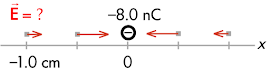## 20160315

### Physics quiz question: electric field of source charge

Physics 205B Quiz 3, spring semester 2016
Cuesta College, San Luis Obispo, CAA –8.0 nC point charge is held at the origin. The magnitude of the electric field at x = –1.0 cm is directed to the __________ and its magnitude is __________.
(A) left; 8.0×10–5 N/C.
(B) left; 7.2×103 N/C.
(C) left; 7.2×105 N/C.
(D) right; 8.0×10–5 N/C.
(E) right; 7.2×103 N/C.
(F) right; 7.2×105 N/C.

Correct answer (highlight to unhide): (F)The direction of the electric field surrounding a negative source charge is inwards, such that at x = –1.0 cm, the electric field points to the right (towards the –8.0 nC source charge). (The magnitudes of these electric field vectors are not quite to scale, but do get smaller with increasing distance from the –8.0 nC source charge).

The magnitude E of the electric field located at a distance r away from a source charge Q is:

E = k·|Q|/r2,

E = (8.99×109 N·m2/C2)·|–8.0×10–9 C|/(0.010 m)2,

E = 719,200 N/C,

or to two significant figures, the magnitude of the electric field at x = –1.0 cm is 7.2×105 N/C.

(Responses (A)-(C) all have the wrong direction; response (D) is Q/r2; respond (E) is k·Q/r.)

Sections 30882, 30883
Exam code: quiz03Ccf7
(A) : 0 students
(B) : 2 students
(C) : 7 students
(D) : 4 students
(E) : 11 students
(F) : 16 students

Success level: 40%
Discrimination index (Aubrecht & Aubrecht, 1983): 0.55